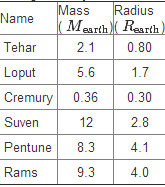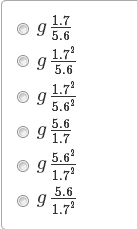# Having problems understanding units

• FTCC.student
I can think of for now).In summary, the universal gravitation constant G is 6.67 x 10-11 N*m2/kg2. This just looks like a mess to me. Newton-meters squared over kilograms squared? I am not sure what this means, and what I can "compare" it to or what I can use to visualize something (weighing this much? Is this a weight?) It was hard to explain my problem here so I am hoping this was coherent.

#### FTCC.student

Sad, right? Problems in Chapter 1! I can usually calculate the correct values, but understanding what the units attached means, I just don't understand the concept...I can't visualize it. For example,

For example, in scientific notation the universal gravitation constant G is this according to my textbook:

G = 6.67 x 10-11 N*m2/kg2

That just looks like a mess to me. Newton-meters squared over kilograms squared? I am not sure what this means, and what I can "compare" it to or what I can use to visualize something (weighing this much? Is this a weight?)

It was hard to explain my problem here so I am hoping this was coherent.

FTCC.student said:
Sad, right? Problems in Chapter 1! I can usually calculate the correct values, but understanding what the units attached means, I just don't understand the concept...I can't visualize it. For example,

For example, in scientific notation the universal gravitation constant G is this according to my textbook:

G = 6.67 x 10-11 N*m2/kg2

That just looks like a mess to me. Newton-meters squared over kilograms squared? I am not sure what this means, and what I can "compare" it to or what I can use to visualize something (weighing this much? Is this a weight?)

It was hard to explain my problem here so I am hoping this was coherent.

$G$ is the proportionality constant in Newton's equation for the force of gravity between two massive bodies.

$|F| = G \frac{M_{1}M_{2}}{|r_{12}|^{2}}$

$F$ is force which is in Newtons $[N]$
$M$ is in kilograms, so $M_{1}M_{2}$ is in $[kg^{2}]$
$r_{12}$ is the distance between masses $M_{1}$ and $M_{2}$ and is in meters $[m]$

$G$ has whatever units it must have to that the units of $F$ are the same as the units of $G \frac{M_{1}M_{2}}{|r_{12}|^{2}}$.

Because the units of $\frac{M_{1}M_{2}}{|r_{12}|^{2}}$ are $[\frac{kg^{2}}{m^{2}}]$, $G$ must have units of $[\frac{N*m^{2}}{kg^{2}}]$ for $F$ to have units of $[N]$.

Hope this helps:)

FTCC.student said:
Sad, right? Problems in Chapter 1! I can usually calculate the correct values, but understanding what the units attached means, I just don't understand the concept...I can't visualize it. For example,

For example, in scientific notation the universal gravitation constant G is this according to my textbook:

G = 6.67 x 10-11 N*m2/kg2

That just looks like a mess to me. Newton-meters squared over kilograms squared? I am not sure what this means, and what I can "compare" it to or what I can use to visualize something (weighing this much? Is this a weight?)

It was hard to explain my problem here so I am hoping this was coherent.

Welcome to the PF.

Handle units just like any algebraic entities, and you will be fine. In fact, carrying units along in equations helps a great deal in finding mistakes in your work. The units of the LHS and RHS of any equation have to be equal, and when adding two variables, their units have to be the same as well.

So write the equation for the gravitational force that uses that G constant above. See how the units of the other quantities cancel out on the RHS, leaving you with the units of force (which matches the units of F on the LHS of the equation...

Does that help?

EDIT -- beaten to the punch by speedy typer jfizzix!argh... "so that"

Look at Newton's law of gravitation: ##F=\frac {GMm}{r^2}##.

We want consistent equations in physics. Something like "1 meter = 1 kg/second" -- that's nonsense. To be a consistent equation, the units on the left hand side of an equality must be the same as those on the right. This tells us what the units of G must be in order to make Newton's law of gravitation a consistent equation. Cast of the numbers from both sides, leaving just the units. Force F -- that's expressed in Newtons. Mass is in kilograms, and length is in meters. Mass*mass has units of kilograms2, length squared has units of meters2.

What we're left with is ##N = \frac{u(G) kg^2}{m^2}##, where ##u(X)## denotes the units in which the quantity is expressed. In this case, we want ##u(G)##. Solve for that by moving all the stuff on the right hand side over to the left-hand side of the equality. It's just like you did way back when in clearing the denominator. Multiplying both sides by ##\frac{m^2}{kg^2}## tells us that ##u(G) = \frac{N\cdot m^2}{kg^2}##.

Let's try that with a variation on Newton's law of gravitation. Combining Newton's law of gravitation with Newton's 2nd law yields ##a=\frac{GM}{r^2}##. Use the same technique as before, one gets that ##u(G) = \frac{m^3}{kg\cdot s^2}##.

So what gives? One way we get ##\frac{N\cdot m^2}{kg^2}##, the other, ##\frac{m^3}{kg\cdot s^2}##. A Newton is a kilogram-meter/second2, so those apparently contradictory sets of units are one and the same. With dimensional analysis it is oftentimes beneficial to use only primitive units, length, mass, time, and charge.

To understand the units, you need to understand the Universal Law of Gravity, where it is used:
##F = G\frac{m_1 m_2}{r^2}##

Note that the m's are masses and the r is a distance. Thus, to get a force (F) you must end up with units of Newton's, thus G must have units that "cancel" the kg^2 from the masses and m^2 from the distance.

Edit: Wow... beaten by three others. I must be sleeping!

Thank you guys so much, very in depth explanations. I do understand where the units in the answer come from, basically just get them algebraically along with the values.

I do have another question regarding mg=GmM/R^2, though

Keep in mind I am only in algebra based physics, and haven't taken a physics class before. Here is the question from Masteringphysics.com:

One day, Punch steps on the bathroom scale and "weighs in" at 236 lb. Punch decides to go to a different planet where he would weigh in at 118 lb. His plan is to travel to Xobing, a newly discovered star with a planetary system. Here is a table listing the planets in that system:In this table, the mass and the radius of each planet are given in terms of the corresponding properties of the earth. For instance, Tehar has a mass equal to 2.1 Earth masses and a radius equal to 0.80 Earth radii.

If acceleration due to gravity on the Earth is g, which formula gives the acceleration due to gravity on Loput?What I'm confused about, is am I supposed to plug in the mass/radius in the mg=GmM/r^2? If so, I am not sure how I am supposed to tell the distance from the fictitious planet to Earth, and I also only see 1 mass (the fict. planet), unless I am supposed to look up what the Earth's mass is too. I am pretty sure the answer of this one is going to have a squared denominator, which narrows me down to choices C, E and F...what should I do?